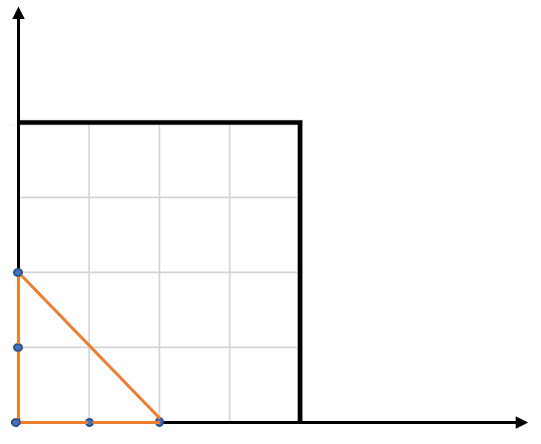812. Largest Triangle Area

You have a list of points in the plane. Return the area of the largest triangle that can be formed by any 3 of the points.

```Example:
Input: points = [[0,0],[0,1],[1,0],[0,2],[2,0]]
Output: 2
Explanation:
The five points are show in the figure below. The red triangle is the largest.
```Notes:

• `3 <= points.length <= 50`.
• No points will be duplicated.
•  `-50 <= points[i][j] <= 50`.
• Answers within `10^-6` of the true value will be accepted as correct.

812. Largest Triangle Area
``````struct Solution;

impl Solution {
fn largest_triangle_area(points: Vec<Vec<i32>>) -> f64 {
let mut res: f64 = 0f64;
for i in &points {
for j in &points {
for k in &points {
let area = (i * j + j * k + k * i
- j * i
- k * j
- i * k)
.abs();
let area = area as f64 / 2f64;
res = f64::max(area, res);
}
}
}
res
}
}

#[test]
fn test() {
use assert_approx_eq::assert_approx_eq;
let points: Vec<Vec<i32>> = vec_vec_i32![[0, 0], [0, 1], [1, 0], [0, 2], [2, 0]];
assert_approx_eq!(Solution::largest_triangle_area(points), 2f64);
}
``````